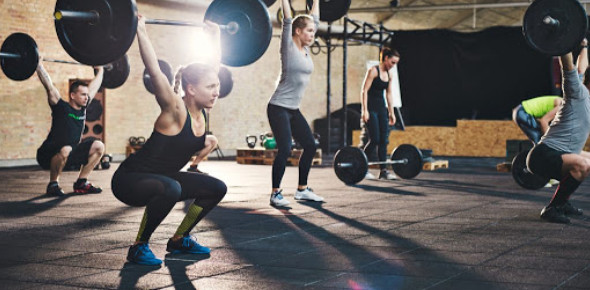# Strength & Conditioning Test

33 Questions | Attempts: 1732
ShareSettingsThis exam will test student knowledge of the concepts learned in the strength and conditioning class.

• 1.
Name the three lower body joints extended during the hang clean.
• 2.
What are the three principles of our strength and conditioning program?
• 3.
A shoulder width stance is used when hang cleaning.
• A.

True

• B.

False

• 4.
A jump shrug is one part of the squat progression.
• A.

True

• B.

False

• 5.
The squat is considered the "King of Exercise".
• A.

True

• B.

False

• 6.
A hip width stance is used when squatting,
• A.

True

• B.

False

• 7.
To gain the full benefits of the squat, an athlete should be squatting to parallel.
• A.

True

• B.

False

• 8.
How much does the Olympic barbell used for the bench press, squat, and clean weigh?
• A.

55 pounds

• B.

45 pounds

• C.

135 pounds

• D.

95

• 9.
Static stretching is performed after the workout.
• A.

True

• B.

False

• 10.
Name five different phase one dynamic warm-up exercises
• 11.
Which of these three is considered a "push" exercise
• A.

Lat pull-down

• B.

One-arm row

• C.

Military press

• 12.
Which of these three is considered a "pull exercise"
• A.

One-arm row

• B.

Military press

• C.

Bench press

• 13.
The agility ladder drills should be performed forward and backwards only.
• A.

True

• B.

False

• 14.
How much does a "plate" weigh?
• A.

35 pounds

• B.

25 pounds

• C.

45 pounds

• D.

55 pounds

• 15.
How much weight is one lifting when there is two plates on each side of the bar?
• A.

135 pounds

• B.

225 pounds

• C.

185 pounds

• 16.
A spotter should be assisting the person who is bench pressing, squatting, and incline bench pressing.
• A.

True

• B.

False

• 17.
The pro-agility drill inolves how many total yards of running.
• A.

10

• B.

20

• C.

15

• D.

25

• E.

5

• 18.
What is the definition of lateral movement?
• A.

To move backwards

• B.

To move forward

• C.

To move sideways

• D.

To jump in the air

• 19.
Plyometrics is also referred to as "jump training".
• A.

True

• B.

False

• 20.
Plyometrics is defined as
• A.

Exercise in which the muscles are repeatedly stretched and suddenly contracted

• B.

The power of moving quickly and easily

• C.

Full, maximum, or optimum rate of motion

• 21.
Agililty is defined as
• A.

Full, maximum, or optimum rate of motion

• B.

how quickly you change direction

• C.

How fast you are

• D.

How high you can jump

• 22.
In a timed dot drill, the five different foot patterns are performed how many times?
• A.

3

• B.

4

• C.

6

• D.

5

• 23.
What are the five different exercises in a timed dot drill?
• 24.
What are the set x reps of Week 1, 2, 3, and 4 of the weight sheets?
• 25.
Name four lower body core lifts

## Related TopicsBack to top
×

Wait!
Here's an interesting quiz for you.